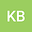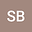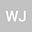Internal Bernstein Functions and Lévy-Laplace exponents
•••• Kholoud Basalim,
• Safa Bridaa,
• Wissem Jedidi
Kholoud Basalim
King Saud University

Corresponding Author:[email protected]

Author ProfileSafa Bridaa
Universite de Tunis El Manar Faculte des Sciences de Tunis
Author ProfileWissem Jedidi
University of Tunis El Manar Department of Mathematics
Author Profile## Abstract

Bertoin, Roynette et Yor \cite{bertion} described new connections between the class $\Bd$ of L\’evy-Laplace exponents $\Psi$ (also called the class (sub)critical branching mechanism) and the class of Bernstein functions ($\BF$) which are internal, i.e. those Bernstein functions $\phi$ s.t. $\Psi \circ \phi$ remains a Bernstein function for every $\Psi$. We complete their work and illustrate how the class f internal function is rich from the stochastic point of view. It is well known that every $\phi \in \BF$ corresponds univocally to: (i) a subordinator ${(X_t)}_{t\geq 0}$ (or equivalently to transition semigroups ${\big(\pr(X_t\in dx)\big)}_{t\geq 0}$; (ii) a L\’evy measure $\mu$ (which controls the jumps of the subordinator). It is also known that, on $\oi$, the measure $\pr(X_t \in dx)/t$ converges vaguely to $\dd \delta_0(dx)+ \mu(dx)$ as $t\to 0$, where $\dd$ is the drift term, but rare are the situations where we can compare the transition semigroups with the L\’evy measure. Our extensive investigations on the composition of L\’evy-Laplace exponents $\Psi$ with Bernstein functions show, for instance, this remarkable facts: $\phi$ is internal is equivalent to: (a) $\phi^2 \in \BF$ or to (b) $t\mu(dx) - \pr(X_t\in dx)$ is a positive measure on $\oi$. We also provide conditions on $\mu$ insuring that $\phi$ is internal. We also show L\’evy-Laplace exponents are closely connected to the class of Thorin Bernstein function and provide conditions on $\mu$ insuring that $\phi$ is internal.
28 Feb 2020Submitted to Mathematical Methods in the Applied Sciences
07 Mar 2020Submission Checks Completed
07 Mar 2020Assigned to Editor
09 Mar 2020Reviewer(s) Assigned
04 Jun 2020Review(s) Completed, Editorial Evaluation Pending
05 Jun 2020Editorial Decision: Revise Minor